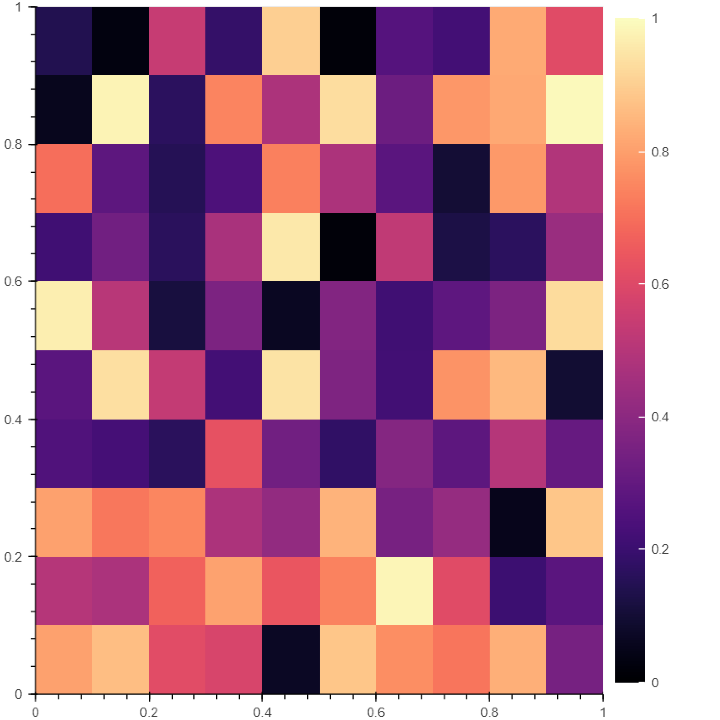# 如何在Bokeh中添加颜色条

## 在Bokeh中添加颜色条的逐步过程

### 示例

# import NumPy library
import numpy as np

# from bokeh.plot import figure and show for creating and displaying plot
from bokeh.plotting import figure, show

# from bokeh.models import LinearColorMapper and ColorBar to generate a color bar
from bokeh.models import LinearColorMapper, ColorBar
from bokeh.io import output_notebook
output_notebook()


### 示例

# Generate a random array of size 10 to plot
data = np.random.rand(10,10)


### 步骤3：决定颜色栏的颜色调色板

# 使用线性颜色映射函数选择栏的颜色调色板

# 指定颜色映射的低值和高值以确定数值范围。

color = LinearColorMapper(palette = "Magma256", low = 0, high = 1)


### 步骤4：定义X和Y轴的限制

# Defining X axis and Y axis limits
plot = figure(x_range = (0, 1), y_range = (0,1))


### 步骤5：生成图表的图像

#Add the plot's width and height, scalar data, and a color mapper to generate a plot image
plot.image(image = [data], color_mapper = color, dh = , dw = , x = , y = )


### 步骤6：创建色条

# Creating the color bar and setting the color bar position at (0,0)
color_bar = ColorBar(color_mapper = color, location = (0,0))


### 第7步：定位颜色条并显示图形

# Incorporating the color bar on the right
# display the plot
show(plot)


### 输出• 回顶